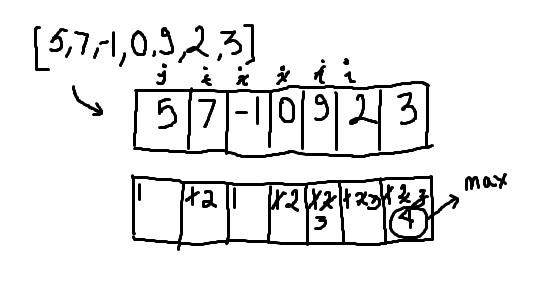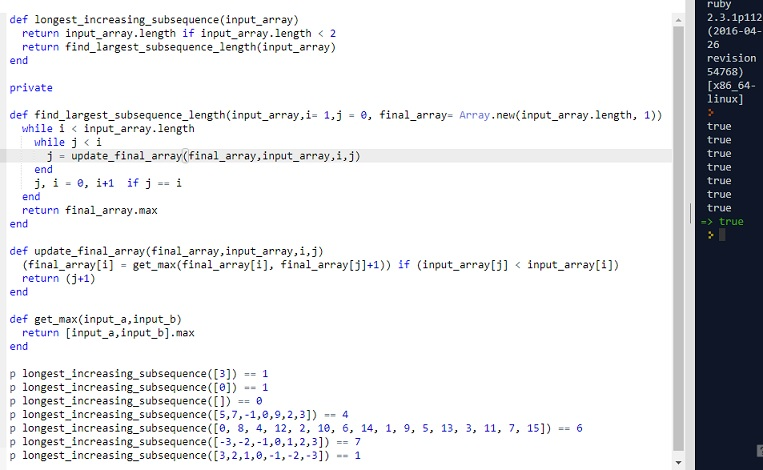# (*) CLEAN CODE: DYNAMIC PROGRAMMING: LONGEST INCREASING SUBSEQUENCE

By: Saurav

2017-11-10 21:14:00 UTC

Find the longest increasing subsequence of a given sequence / array.

In other words, find a subsequence of array in which the subsequence’s elements are in strictly increasing order, and in which the subsequence is as long as possible.
This subsequence is not necessarily contiguous, or unique.
In this case, we only care about the length of the longest increasing subsequence.

Example :

Input : [0, 8, 4, 12, 2, 10, 6, 14, 1, 9, 5, 13, 3, 11, 7, 15]
Output : 6
The sequence : [0, 2, 6, 9, 13, 15] or [0, 4, 6, 9, 11, 15] or [0, 4, 6, 9, 13, 15]
InterviewBit

This is an interesting one!
We need to use dynamic programming to solve this problem.

Since we need increasing sub sequence which may not be contiguous, we need to keep track of the increasing sub sequence of elements till that element and then decide for the current one if its increasing or not.

Figure below help us visualize this.

A great tutorial is available by Tushar Roy

```p longest_increasing_subsequence() == 1
p longest_increasing_subsequence() == 1
p longest_increasing_subsequence([5,7,-1,0,9,2,3]) == 4
p longest_increasing_subsequence([0, 8, 4, 12, 2, 10, 6, 14, 1, 9, 5, 13, 3, 11, 7, 15]) == 6
p longest_increasing_subsequence([-3,-2,-1,0,1,2,3]) == 7
p longest_increasing_subsequence([3,2,1,0,-1,-2,-3]) == 1```As in the figure above, we start by analyzing maximum length of the sub sequence of an array of length 1: 
Then we analyze for array of two elements: [5,7] and store the result in another array.
We do this process again and again for all the elements in the given array and put the maximum number in a final_array.

The algorithm is:

```1. make a final_array, same size as the input_array. Fill it with 1s
2. Initialize two looping variables i and j. i=1, j=0
3. while i < input_array length
while j < i
if input_array[j] < input_array[i]
final_array[i] = [final_array[i], final_array[j]+1].max
if j == i
reset j to 0
increase i
return max of final_array
```

1st Attempt

```def longest_increasing_subsequence(input_array)
return input_array.length if input_array.length < 2
final_array = Array.new(input_array.length, 1)
i,j = 1,0
while i < input_array.length
while j < i
if input_array[j] < input_array[i]
final_array[i] = [final_array[i], final_array[j]+1].max
# p "#{i} index: |#{final_array[i]}|"
end
j+= 1
end
if j == i
j =0
i = i+1
end
end
return final_array.max
end
```

Not so great! Lets work on it a little and make it cleaner, and let function do just one thing.

Attempt 2:

```def longest_increasing_subsequence(input_array)
return input_array.length if input_array.length < 2
return find_largest_subsequence_length(input_array)
end

#private

def find_largest_subsequence_length(input_array,i= 1,j = 0, final_array= Array.new(input_array.length, 1))
while i < input_array.length
while j < i
j = update_final_array(final_array,input_array,i,j)
end
j, i = 0, i+1  if j == i
end
return final_array.max
end

def update_final_array(final_array,input_array,i,j)
(final_array[i] = get_max(final_array[i], final_array[j]+1)) if (input_array[j] < input_array[i])
return (j+1)
end

def get_max(input_a,input_b)
return [input_a,input_b].max
end ```All our tests pass and the code looks clean but its still a O(n^2) solution.

I will update the post after working on a more efficient solution.

### Let me know what you think! twitter: sprakash24octlinkedin

Owned & Maintained by Saurav Prakash

If you like what you see, you can help me cover server costs or buy me a cup of coffee though donation :)